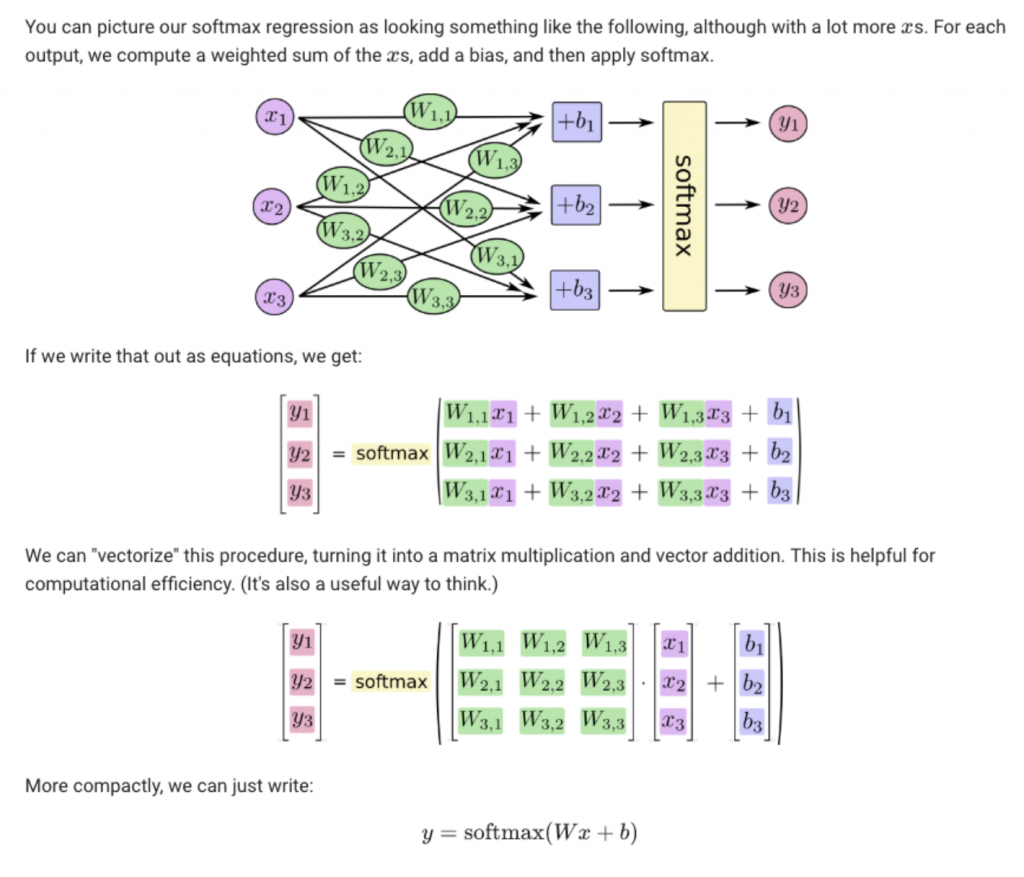2019 iT 邦幫忙鐵人賽DAY 23
0

[第23天] Tensorflow MNIST練習(1)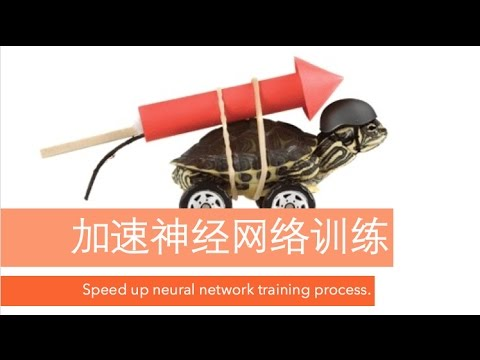https://images2018.cnblogs.com/blog/1192699/201803/1192699-20180311110108768-2113908893.gif
https://images2018.cnblogs.com/blog/1192699/201803/1192699-20180311105558593-251578131.gif

MNIST 變識練習

MNIST是機器學習裡面非常重要的手寫數字資料集，如今車牌變識已經如此廣泛!這裡參考R 語言使用者的 Python 學習筆記tensorflow學習筆記莫凡的PYTHON跟著做一遍~

MNIST 資料集分成三個部分
55,000 筆的 training data (mnist.train)
10,000 筆的 test data (mnist.test)
5,000 筆的 validation data (mnist.validation)

``````from tensorflow.examples.tutorials.mnist import input_data
import numpy as np

# 讀入 MNIST
mnist = input_data.read_data_sets("MNIST_data/", one_hot = True)
x_train = mnist.train.images
y_train = mnist.train.labels
x_test = mnist.test.images
y_test = mnist.test.labels

# 檢視結構
print(x_train.shape)
print(y_train.shape)
print(x_test.shape)
print(y_test.shape)
print("---")

# 檢視一個觀測值
#print(x_train[1, :])
print(np.argmax(y_train[1, :])) # 第一張訓練圖片的真實答案

#3
``````

``````from tensorflow.examples.tutorials.mnist import input_data
import numpy as np
import matplotlib.pyplot as plt

%matplotlib inline

# 讀入 MNIST
mnist = input_data.read_data_sets("MNIST_data/", one_hot = True)
x_train = mnist.train.images

# 印出來看看
first_train_img = np.reshape(x_train[1, :], (28, 28))
plt.matshow(first_train_img, cmap = plt.get_cmap('gray'))
plt.show()
``````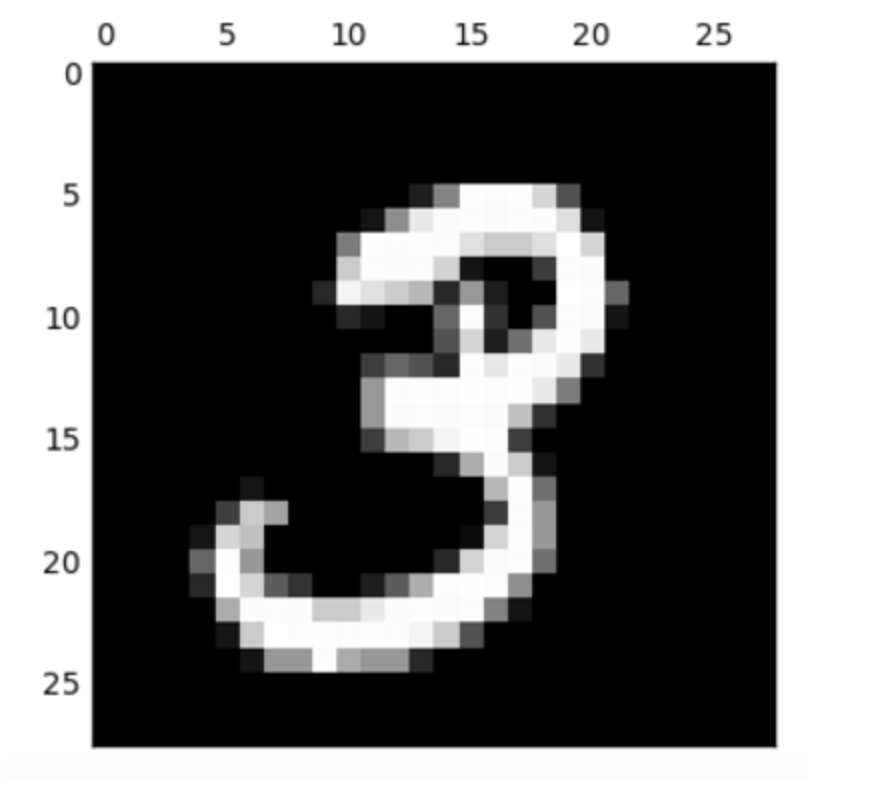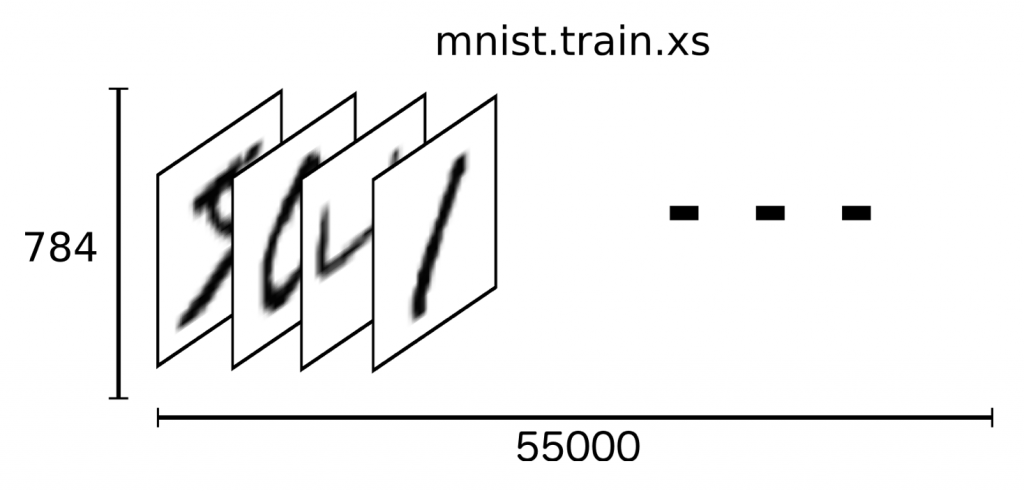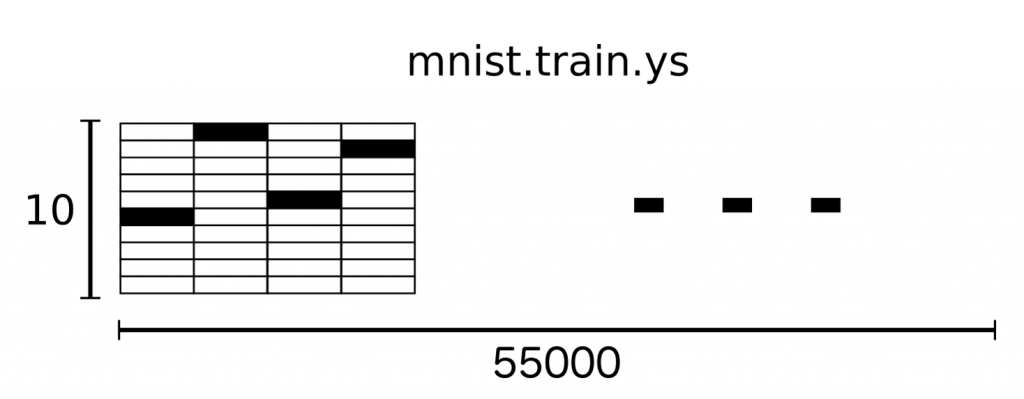Softmax